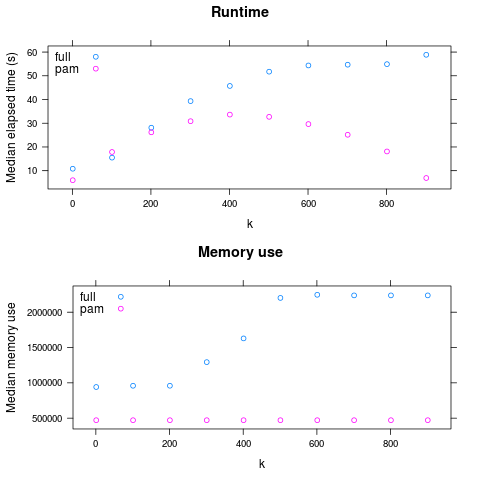# Comparing two algorithms¶

This is a realistic example of using `nestly` to examine the performance of two algorithms. Source code to run it is available in `examples/adcl/`.

We will use the `min_adcl_tree` subcommand of the `rppr` tool from the `pplacer` suite, available from http://matsen.fhcrc.org/pplacer.

This tool chooses `k` representative leaves from a phylogenetic tree. There are two implementations: the Full algorithm solves the problem exactly, while the PAM algorithm uses a variation on the partitioning among medoids heuristic to find a solution.

We’d like to compare the two algorithms on a variety of trees, using different values for `k`.

## Making the nest¶

Setting up the comparison is demonstrated in `00make_nest.py`, which builds up combinations of `(algorithm, tree, k)`:

 ``` 1 2 3 4 5 6 7 8 9 10 11 12 13 14 15 16 17 18 19 20 21 22 23 24 25 26 27 28 29 30 31 32 33 34 35 36 37 38 39 40 41 42 43 44 45 46 47``` ```#!/usr/bin/env python # This example compares runtimes of two implementations of # an algorithm to minimize the average distance to the closest leaf # (Matsen et. al., accepted to Systematic Biology). # # To run it, you'll need the `rppr` binary on your path, distributed as part of # the pplacer suite. Source code, or binaries for OS X and 64-bit Linux are # available from http://matsen.fhcrc.org/pplacer/. # # The `rppr min_adcl_tree` subcommand takes a tree, an algorithm name, and # the number of leaves to keep. # # We wish to explore the runtime, over each tree, for various leaf counts. import glob from os.path import abspath from nestly import Nest, stripext # The `trees` directory contains 5 trees, each with 1000 leaves. # We want to run each algorithm on all of them. trees = [abspath(f) for f in glob.glob('trees/*.tre')] n = Nest() # We'll try both algorithms n.add('algorithm', ['full', 'pam']) # For every tree n.add('tree', trees, label_func=stripext) # Store the number of leaves - always 1000 here n.add('n_leaves', , create_dir=False) # Now we vary the number of leaves to keep (k) # Sample between 1 and the total number of leaves. def k(c): n_leaves = c['n_leaves'] return range(1, n_leaves, n_leaves // 10) # Add `k` to the nest. # This will call k with each combination of (algorithm, tree, n_leaves). # Each value returned will be used as a possible value for `k` n.add('k', k) # Build the nest: n.build('runs') ```

Running that:

```\$ ./00make_nest.py
```

Creates a new directory, `runs`.

Within this directory are subdirectories for each algorithm:

```runs/full
runs/pam
```

Each of these contains a directory for each tree used:

```\$ ls runs/pam
random001  random002  random003  random004  random005
```

Within each of these subdirectories are directories for each choice of `k`.

```\$ ls runs/pam/random001
1  101  201  301  401  501  601  701  801  901
```

These directories are leaves. There is a JSON file in each, containing the choices made. For example, `runs/full/random003/401/control.json` contains:

```{
"algorithm": "full",
"n_leaves": 1000,
"k": 401
}
```

## Running the algorithm¶

The `nestrun` command-line tool allows you to run a command for each combination of parameters in a nest. It allows you to substitute parameters chosen by surrounding them in curly brackets, e.g. `{algorithm}`.

To see how long, and how much memory each run uses, we’ll use the short shell script `time_rppr.sh`:

 ```1 2 3 4 5 6``` ```#!/bin/sh export TIME='elapsed,maxmem,exitstatus\n%e,%M,%x' /usr/bin/time -o time.csv \ rppr min_adcl_tree --algorithm {algorithm} --leaves {k} {tree} ```

Note the placeholders for the parameters to be provided at runtime: `k`, `tree`, and `algorithm`.

Running a script like `time_rppr.sh` on every experiment within a nest in parallel is facilitated by the `nestrun` script distributed with `nestly`:

```\$ nestrun -j 4 --template-file time_rppr.sh -d runs
```

(this will take awhile)

This command runs the shell script `time_rppr.sh` for each parameter choice, substituting the appropriate parameters. The `-j 4` flag indicates that 4 processors should be used.

## Aggregating results¶

Now we have a little CSV file in each leaf directory, containing the running time:

```|----------+--------+-------------|
|  elapsed | maxmem | exitstatus  |
|----------+--------+-------------|
|  17.78   | 471648 | 0           |
|----------+--------+-------------|
```

To analyze these en-masse, we need to combine them and add information about the parameters used to generate them. The `nestagg` script does just this.

```\$ nestagg delim -d runs -o results.csv time.csv -k algorithm,k,tree
```

Where `-d runs` indicates the directory containing program runs; ```-o results.csv``` specifies where to write the output; `time.csv` gives the name of the file in each leaf directory, and `-k algorithm,k,tree` lists the parameters to add to each row of the CSV files.

Looking at `results.csv`:

```|----------+---------+------------+-----------+---------------------------------------+------|
|  elapsed | maxmem  | exitstatus | algorithm | tree                                  | k    |
|----------+---------+------------+-----------+---------------------------------------+------|
|  17.04   | 941328  | 0          | full      | .../examples/adcl/trees/random001.tre | 1    |
|  20.86   | 944336  | 0          | full      | .../examples/adcl/trees/random001.tre | 101  |
|  31.75   | 944320  | 0          | full      | .../examples/adcl/trees/random001.tre | 201  |
|  39.34   | 980048  | 0          | full      | .../examples/adcl/trees/random001.tre | 301  |
|  37.84   | 1118960 | 0          | full      | .../examples/adcl/trees/random001.tre | 401  |
|  42.15   | 1382000 | 0          | full      | .../examples/adcl/trees/random001.tre | 501  |
etc
```

Now we have something we can look at!So: PAM is faster for large `k`, and always has lower maximum memory use.

(generated by `examples/adcl/03analyze.R`)